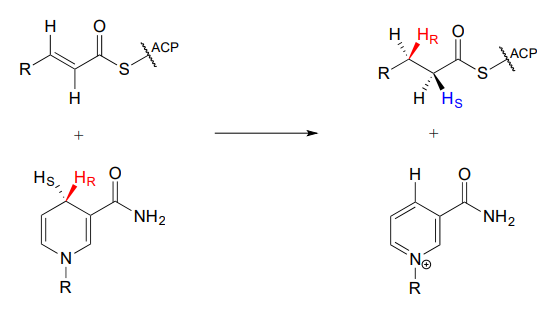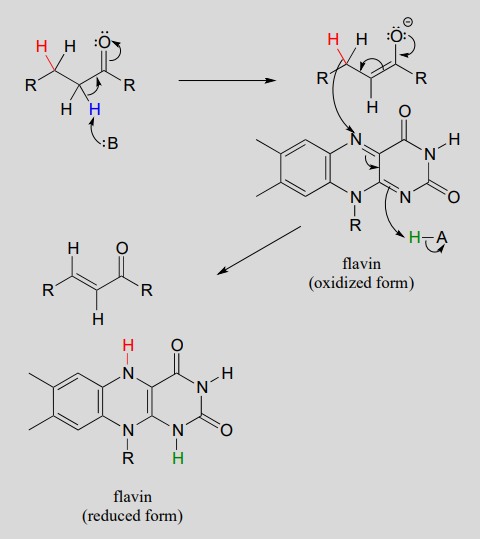# 11.5: Hydrogenation of alkenes and Dehydrogenation of Alkanes

$$\newcommand{\vecs}{\overset { \rightharpoonup} {\mathbf{#1}} }$$ $$\newcommand{\vecd}{\overset{-\!-\!\rightharpoonup}{\vphantom{a}\smash {#1}}}$$$$\newcommand{\id}{\mathrm{id}}$$ $$\newcommand{\Span}{\mathrm{span}}$$ $$\newcommand{\kernel}{\mathrm{null}\,}$$ $$\newcommand{\range}{\mathrm{range}\,}$$ $$\newcommand{\RealPart}{\mathrm{Re}}$$ $$\newcommand{\ImaginaryPart}{\mathrm{Im}}$$ $$\newcommand{\Argument}{\mathrm{Arg}}$$ $$\newcommand{\norm}{\| #1 \|}$$ $$\newcommand{\inner}{\langle #1, #2 \rangle}$$ $$\newcommand{\Span}{\mathrm{span}}$$ $$\newcommand{\id}{\mathrm{id}}$$ $$\newcommand{\Span}{\mathrm{span}}$$ $$\newcommand{\kernel}{\mathrm{null}\,}$$ $$\newcommand{\range}{\mathrm{range}\,}$$ $$\newcommand{\RealPart}{\mathrm{Re}}$$ $$\newcommand{\ImaginaryPart}{\mathrm{Im}}$$ $$\newcommand{\Argument}{\mathrm{Arg}}$$ $$\newcommand{\norm}{\| #1 \|}$$ $$\newcommand{\inner}{\langle #1, #2 \rangle}$$ $$\newcommand{\Span}{\mathrm{span}}$$$$\newcommand{\AA}{\unicode[.8,0]{x212B}}$$

We turn next to reactions in which a hydrogen molecule is added to the double bond of an alkene, forming an alkane - and the reverse, in which $$H_2$$ is eliminated from an alkane to form an alkene. Many biochemical reactions of this type involve $$\alpha$$, $$\beta$$-unsaturated thioesters.## Alkene hydrogenation

In the cell, alkene hydrogenation most often occurs at the a and b position relative to a carbonyl. This type of alkene hydrogenation is essentially a conjugate addition (section 11.4) of hydrogen, with a hydride ion (often from $$NAD(P)H$$) acting as the nucleophile in the first step.

$$NAD(P)H$$-dependent hydrogenation (reduction) of an $$\alpha$$, $$\beta$$-conjugated alkene:Mechanism:As part of the fatty acid synthesis pathway, a double bond between the a and b carbons of a fatty acid is reduced to a single bond by hydrogenation (EC 1.3.1.10). The fatty acid is attached to an acyl-carrier protein via a thioester linkage (section 11.5).It can be easy to forget but important to remember that there is a lot of stereospecificity inherent in biochemical reactions, including this one - even though no chiral centers are involved. First, notice that the substrate contains a trans (E) alkene. Next, let's add some new information about prochirality:Notice that in this particular reaction it is specifically the pro-R hydride on $$NADPH$$ that is delivered to the substrate. Also notice that the hydride and proton are added to the same side of the alkene, and become the pro-R and pro-S hydrogen atoms, respectively, on the substrate. This level of stereospecificity, you should recall from previous discussions, stems from the highly precise positioning of substrate and cofactor within the active site of the enzyme.

Other hydrogenase enzymes are known to deliver the pro-S hydride of $$NADH$$ or $$NADPH$$ to their substrate, and there are many examples of biochemical conjugate addition reactions in which the nucleophile and proton are added from opposite sides. Always keep in mind that stereochemistry is a key element in the amazing diversity of biological organic reactions.

## Flavin-dependent alkane dehydrogenation

Next let's consider an alkane dehydrogenation reaction (EC 1.3.99.3) in the fatty acid degradation pathway. Here, a double bond is introduced between the $$\alpha$$ and $$\beta$$ carbons, with concurrent loss of a hydride ion and a proton.This reaction is clearly not the reverse of the hydrogenation reaction we just saw from fatty acid biosynthesis. First of all, you should notice that the thioester linkage is to coenzyme A rather than acyl carrier protein ($$ACP$$). More importantly to this discussion, while the hydride donor in the biosynthetic hydrogenation reaction is $$NADPH$$, the relevant coenzyme in the catabolic direction is not $$NAD^+$$ or $$NADP^+$$ - rather, it is a flavin coenzyme.

Flavin adenine dinucleotide ($$FAD$$) is composed of three components: the three-ring flavin system, ribose phosphate, and AMP. An alternate form, which is missing the AMP component, is called flavin mononucleotide ($$FMN$$).The reactive part of the coenzyme is the flavin group, so usually the rest of the molecule is abbreviated with 'R'.

$$FAD$$ and $$FMN$$ are the oxidized form of flavin. The reduced (hydrogenated) forms of these cofactors are abbreviated $$FADH_2$$ and $$FMNH_2$$.The flavin coenzymes are synthesized in humans from riboflavin (vitamin $$B_2$$), which we obtain from our diet (the structure of riboflavin is the same as that of $$FMN$$, except that riboflavin lacks the phosphate group). Notice the extended conjugated p system in the three fused rings: the flavin system absorbs light in the visible wavelengths and has a distinctive deep yellow color - it is riboflavin, and to some extent $$FAD$$ and $$FMN$$, that give urine its color.

Like the nicotinamide coenzymes, flavin serves as a hydride donor or acceptor. $$FAD$$ and $$FMN$$ are able to accept a hydride ion (and a proton), and $$FADH_2$$ and $$FMNH_2$$ in turn can serve as hydride donors in hydrogenation reactions.Below is a general mechanism for the dehydration of an alkene at the $$\alpha$$, $$\beta$$ position - notice that it is mechanistically an E1cb elimination of $$H_2$$.

$$\alpha$$, $$\beta$$ dehydrogenation (oxidation) of an alkane:Mechanism:In many enzymatic reactions in which $$FADH_2$$ acts as the reducing agent, the reaction cycle is completed when $$FAD$$, rather than being released from the active site, is recycled back to $$FADH_2$$ with the concomitant oxidation of $$NADH$$.Hydride ion transfer with flavin or nicotinamide coenzymes is a two electron redox process. However, unlike the nicotinamide cofactors, flavins are also able to function in single electron transfer (radical) mechanisms. We will come back to this idea briefly in the chapter 16.

##### Exercise 15.5.1

Fumarate is formed in an alkane dehydrogenation reaction (EC 1.3.5.1) which is part of the citric acid cycle:1. Predict the structure of the starting substrate in this reaction
2. Draw the structure of the enolate intermediate
##### Exercise 15.5.2

Reduced flavin can serve as the hydride donor in some hydrogenation reactions. Degradation of the RNA base uracil begins with hydrogenation of a conjugated alkene group by a flavin-dependent hydrogenase enzyme (EC 1.3.1.2). Predict the product of this step, and draw curved arrows for the first mechanistic step.

This page titled 11.5: Hydrogenation of alkenes and Dehydrogenation of Alkanes is shared under a CC BY-NC-SA 4.0 license and was authored, remixed, and/or curated by Tim Soderberg.